Home MonkeyNotes Printable Notes Digital Library Study Guides Study Smart Parents Tips College Planning Test Prep Fun Zone Help / FAQ How to Cite New Title Request

4.3 Derivability and Continuity of A Function At A Point

THEOREM: If a function f  is derivable at x, then f  is continuous at x.

PROOF: We are given that f is derivable at x

\ f  (x) =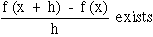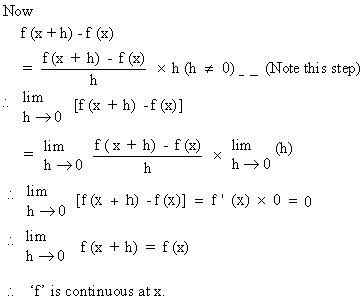Your browser does not support the IFRAME tag.

REMARK: The converse of this theorem i.e. " If f  is continuous at x , then f is derivable at x " is not true. This can be seen from the following examples.

Example 3

Shows that the function f defined by f(x) = |x| is continuous but not derivable at x = 0.

We have f (0) = 0 and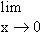|x| = 0. Hencef (x) = f (0) and f  is continuous at x = 0.

From Example 1 we have already proved that f  is not derivable at x = 0

Example 4

If f (x) = x sin, x ¹ 0 and f (0) = 0, show that f  is continuous but not derivable at x = 0.

We have f (0) = 0 andx sin= (a finite limit) ´ 0 = 0 since sinalways lies between -1 and 1 for any value of x.
Therefore -|x|
£ x sin£ |x|

But|x| = 0 and- |x| = 0 Þx sin= 0

Now  f "(0) =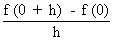=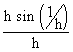, for the given function.

\ f  (0) =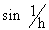which does not exist, Hence f  is not derivable at x = 0

Index

4. 1 Derivability At A Point
4. 2 Derivability In An Interval
4. 3 Derivability And Continuity Of A Function At A Point
4. 4 Some Counter Examples
4. 5 Interpretation Of Derivatives
4. 6 Theorems On Derivatives (differentiation Rules)
4. 7 Derivatives Of Standard Functions
4. 8 Derivative Of A Composite Function
4. 9 Differentiation Of Implicit Functions
4.10 Derivative Of An Inverse Function
4.11 Derivatives Of Inverse Trigonometric Functions
4.12 Derivatives Of Exponential & Logarithmic Functions
4.13 Logarithmic Differentiation
4.14 Derivatives Of Functions In Parametric Form
4.15 Higher order Derivatives

Chapter 5